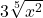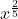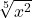Question

Write 3 times x to the two fifths power in radical form. 3 times the fifth root of x squared the fifth root of quantity 3 times x squared 3 times the square root of x to the fifth power the square root of quantity 3 times x to the fifth power

1.bonexptipStep-by-step explanation:

2.phucdien

3 times the fifth root of x squared

Step-by-step explanation:

Write 3 times x to the two fifths power in radical form.

A radical form is a situation where we have to represent a number in the form

3 ×= 3 ×